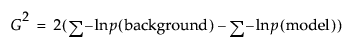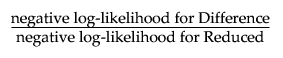Basic Analysis > Logistic Analysis > Statistical Details for the Logistic Platform
Publication date: 11/10/2021

# Statistical Details for the Logistic Platform

This section contains statistical details for the Whole Model Test report.

#### Chi-Square

The Chi-Square statistic is sometimes denoted G2 and is defined as follows:where the summations are over all observations instead of all cells.

#### RSquare (U)

The ratio of this test statistic to the background log-likelihood is subtracted from 1 to calculate R2. More simply, RSquare (U) is computed as follows:using quantities from the Whole Model Test report.

Note: RSquare (U) is also known as McFadden’s pseudo R-square.